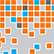# Online Program

Friday, May 31The Cutting Edge in Statistical Machine Learning
Fri, May 31, 1:30 PM - 3:05 PM
Regency Ballroom AB

### Two-Stage Computational Framework for Sparse Generalized Eigenvalue Problem (305045)

*Kean Ming Tan, University of Minnesota

Keywords: Convex relaxation, non-convex optimization, sufficient dimension reduction, Fisher's discriminant analysis, canonical correlation analysis

Sparse generalized eigenvalue problem (GEP) plays a pivotal role in a large family of high-dimensional learning tasks, including sparse Fisher’s discriminant analysis, canonical correlation analysis, and sufficient dimension reduction. Most of the existing methods and theory in the context of specific statistical models that can be recast into sparse GEP require restrictive structural assumptions on the input matrices. This talk will focus on a two-stage computational framework for solving the sparse GEP. At the first stage, we solve a convex relaxation of the sparse GEP. Taking the solution as an initial value, we then exploit a non-convex optimization perspective and propose the truncated Rayleigh flow method (Rifle) to estimate the leading generalized eigenvector, and show that it converges linearly to a solution with the optimal statistical rate of convergence. Theoretically, our method significantly improves upon the existing literature by eliminating the structural assumptions on the input matrices. Numerical studies in the context of several statistical models are provided to validate the theoretical results. We then apply the proposed method on an electrocorticography dataset to understand how human brains recall sequences of words.